# geoplot

Plot line in geographic coordinates

Since R2018b

•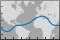## Syntax

``geoplot(lat,lon)``
``geoplot(lat,lon,LineSpec)``
``geoplot(lat1,lon1,...,latN,lonN)``
``geoplot(lat1,lon1,LineSpec1,...,latN,lonN,LineSpecN)``
``geoplot(tbl,latvar,lonvar)``
``geoplot(ax,___)``
``geoplot(___,Name,Value)``
``h = geoplot(___)``

## Description

### Vector Data

example

````geoplot(lat,lon)` plots a line in geographic coordinates. Specify latitude coordinates in degrees using `lat`, and specify longitude coordinates in degrees using `lon`. If the current axes is not a geographic or map axes, or if there is no current axes, then the function plots the line in a new geographic axes.```

example

````geoplot(lat,lon,LineSpec)` creates the plot using the specified line style, marker symbol, and color.```

example

````geoplot(lat1,lon1,...,latN,lonN)` plots pairs of latitude and longitude coordinates using multiple lines on the same axes.```
````geoplot(lat1,lon1,LineSpec1,...,latN,lonN,LineSpecN)` specifies the line style, marker symbol, and color for each line. You can specify `LineSpec` for some lines and omit it for others. For example, `geoplot(lat1,lon1,"o",lat2,lon2)` specifies markers for the first line but not for the second line.```

### Table Data

example

````geoplot(tbl,latvar,lonvar)` plots the variables `latvar` and `lonvar` from the table `tbl`. To plot one data set, specify one variable for `latvar` and one variable for `lonvar`. To plot multiple data sets, specify multiple variables for `latvar`, `lonvar`, or both. If both arguments specify multiple variables, they must specify the same number of variables. (Since R2022b) ```

````geoplot(ax,___)` displays the plot in the axes specified by `ax`. Specify the axes as the first argument in any of the previous syntaxes.```

example

````geoplot(___,Name,Value)` specifies properties of the chart line using one or more name-value arguments. The properties apply to all the plotted lines. For a list of properties, see Line Properties.```
````h = geoplot(___)` returns a column vector of chart line objects. Each object corresponds to a plotted line. Use `h` to modify the properties of the plot after creating it. For a list of properties, see Line Properties. NoteMapping Toolbox™ extends the functionality of the `geoplot` (MATLAB®) function. It adds support for displaying points, lines, and polygons with coordinates in any supported geographic or projected coordinate reference system (CRS). For the `geoplot` (Mapping Toolbox) page, see `geoplot` (Mapping Toolbox). ```

## Examples

collapse all

Load a MAT file containing coordinates for the perimeter of the contiguous United States. The variables within the MAT file, `uslat` and `uslon`, specify latitude and longitude coordinates, respectively, in degrees. Display the coordinates over a topographic basemap.

```load usapolygon.mat geoplot(uslat,uslon) geobasemap topographic```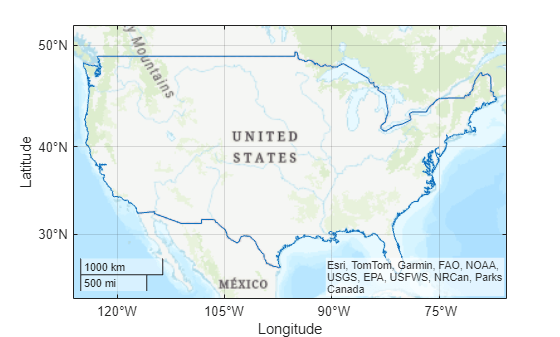Load a MAT file containing coordinates for each contiguous US state. Extract the coordinates for Indiana, Tennessee, and West Virginia.

```load usastates.mat lat1 = usastates(12).Lat; lon1 = usastates(12).Lon; lat2 = usastates(40).Lat; lon2 = usastates(40).Lon; lat3 = usastates(46).Lat; lon3 = usastates(46).Lon;```

Display the state outlines using three lines.

`geoplot(lat1,lon1,lat2,lon2,lat3,lon3)`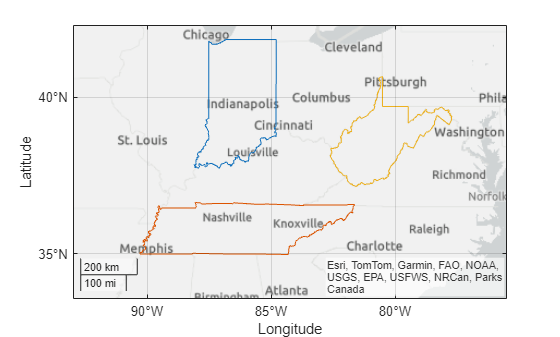Specify the latitude and longitude coordinates of several points along a highway in Germany. Display the points as a line over the `streets` basemap using a black dashed line with circle markers.

```lat = [48.915 48.907 48.901 48.893 48.887 48.881 48.875 48.869 48.865]; lon = [10.192 10.188 10.182 10.171 10.166 10.165 10.167 10.173 10.184]; geoplot(lat,lon,"k--o") geobasemap streets```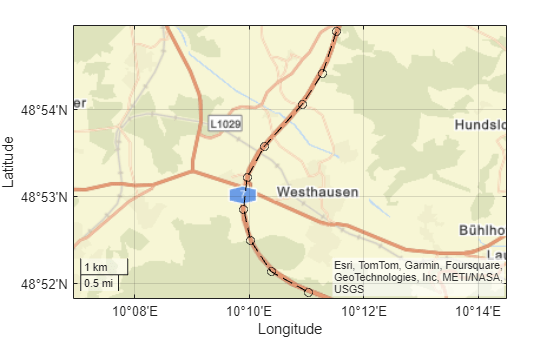Specify the latitude and longitude coordinates of several points along the Mississippi River.

```lat = [32.30 33.92 35.17 36.98 37.69 38.34]; lon = [-91.05 -91.18 -90.09 -89.11 -89.52 -90.37];```

Create a line plot from the data. Use the `LineSpec` option to specify a dashed green line with square markers. Use name-value arguments to specify the line width, marker size, and marker colors.

```geoplot(lat,lon,"--gs","LineWidth",2, ... "MarkerSize",10,"MarkerEdgeColor","b", ... "MarkerFaceColor",[0.5 0.5 0.5])```

Zoom out by adjusting the latitude and longitude limits of the plot. Then, change the basemap to a terrain basemap.

```geolimits([31.60 38.91],[-95.61 -84.27]) geobasemap grayterrain```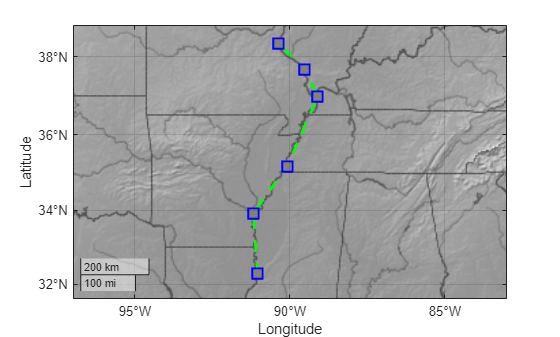Specify the geographic coordinates and names of several places in Boston.

```lat = [42.3501 42.3598 42.3626 42.3668 42.3557]; lon = [-71.0870 -71.0662 -71.0789 -71.0801 -71.0662]; n = [" BACK BAY"," BEACON HILL"," BROAD CANAL"," EAST CAMBRIDGE"," FLAGSTAFF HILL"];```

Plot the locations using black star markers over the `streets` basemap. Zoom out of the plot by adjusting the limits.

```geoplot(lat,lon,"k*") geobasemap streets geolimits([42.3456 42.3694],[-71.0930 -71.0536])```

Display the place names at the same locations.

`text(lat,lon,n)`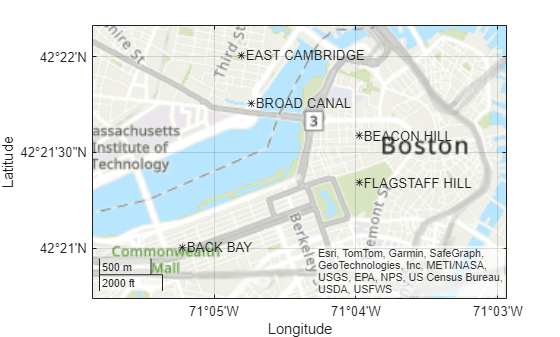Since R2022b

A convenient way to plot data from a table is to pass the table to the `geoplot` function and specify the variables to plot.

Load a MAT file containing cyclone data into the workspace. Create a table using a subset of the data. The table includes latitude and longitude coordinates in the table variables `Latitude` and `Longitude`, respectively.

```load cycloneTracks tbl = cycloneTracks(cycloneTracks.ID == 1320,:);```

Plot the latitude and longitude coordinates over a topographic basemap. Return the `Line` object as `h`.

```h = geoplot(tbl,"Latitude","Longitude"); geobasemap topographic```

Change the marker style and color of the plot by setting the `Marker` and `Color` properties.

```h.Marker = "."; h.Color = "m";```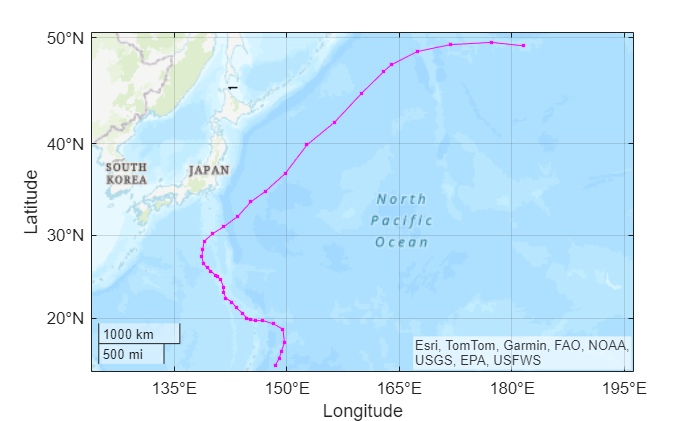## Input Arguments

collapse all

Latitude coordinates in degrees, specified as a vector.

Create breaks in the lines using `NaN` values. For example, this code plots the first two elements, skips the third element, and then plots another line using the last two elements.

```geoplot([10 20 NaN 30 40],[10 20 NaN 30 40]) ```

The sizes of `lat` and `lon` must match.

Example: `[43.0327 38.8921 44.0435]`

Data Types: `single` | `double`

Longitude coordinates in degrees, specified as a vector.

Create breaks in the lines using `NaN` values. For example, this code plots the first two elements, skips the third element, and then plots another line using the last two elements.

```geoplot([10 20 NaN 30 40],[10 20 NaN 30 40]) ```

The sizes of `lat` and `lon` must match.

Example: `[-107.5556 -77.0269 -72.5565]`

Data Types: `single` | `double`

Line style, marker, and color, specified as a string scalar or character vector containing symbols. The symbols can appear in any order. You do not need to specify all three characteristics (line style, marker, and color). For example, if you omit the line style and specify the marker, then the plot shows only the marker and no line.

Example: `"--or"` is a red dashed line with circle markers.

Line StyleDescriptionResulting Line
`"-"`Solid line`"--"`Dashed line`":"`Dotted line`"-."`Dash-dotted lineMarkerDescriptionResulting Marker
`"o"`Circle`"+"`Plus sign`"*"`Asterisk`"."`Point`"x"`Cross`"_"`Horizontal line`"|"`Vertical line`"square"`Square`"diamond"`Diamond`"^"`Upward-pointing triangle`"v"`Downward-pointing triangle`">"`Right-pointing triangle`"<"`Left-pointing triangle`"pentagram"`Pentagram`"hexagram"`HexagramColor NameShort NameRGB TripletAppearance
`"red"``"r"``[1 0 0]``"green"``"g"``[0 1 0]``"blue"``"b"``[0 0 1]``"cyan"` `"c"``[0 1 1]``"magenta"``"m"``[1 0 1]``"yellow"``"y"``[1 1 0]``"black"``"k"``[0 0 0]``"white"``"w"``[1 1 1]`Source table containing the data to plot, specified as a table or a timetable.

Table variables containing the latitude coordinates, specified using one of the indexing schemes from the table.

Indexing SchemeExamples

Variable names:

• `"A"` or `'A'` — A variable called `A`

• `["A","B"]` or `{'A','B'}` — Two variables called `A` and `B`

• `"Var"+digitsPattern(1)` — Variables named `"Var"` followed by a single digit

Variable index:

• An index number that refers to the location of a variable in the table.

• A vector of numbers.

• A logical vector. Typically, this vector is the same length as the number of variables, but you can omit trailing `0` or `false` values.

• `3` — The third variable from the table

• `[2 3]` — The second and third variables from the table

• `[false false true]` — The third variable

Variable type:

• `vartype("categorical")` — All the variables containing categorical values

Regardless of variable names, the axis label on the plot is always `Latitude`.

The variables must contain numeric data of type `single` or `double`. The data must be in the range (–90, 90).

If `latvar` and `lonvar` both specify multiple variables, the number of variables must be the same.

Example: `geoplot(tbl,["lat1","lat2"],"lon")` specifies the table variables named `lat1` and `lat2` for the latitude coordinates.

Example: `geoplot(tbl,2,"lon")` specifies the second variable for the latitude coordinates.

Example: `geoplot(tbl,vartype("numeric"),"lon")` specifies all numeric variables for the latitude coordinates.

Table variables containing the longitude coordinates, specified using one of the indexing schemes from the table.

Indexing SchemeExamples

Variable names:

• `"A"` or `'A'` — A variable called `A`

• `["A","B"]` or `{'A','B'}` — Two variables called `A` and `B`

• `"Var"+digitsPattern(1)` — Variables named `"Var"` followed by a single digit

Variable index:

• An index number that refers to the location of a variable in the table.

• A vector of numbers.

• A logical vector. Typically, this vector is the same length as the number of variables, but you can omit trailing `0` or `false` values.

• `3` — The third variable from the table

• `[2 3]` — The second and third variables from the table

• `[false false true]` — The third variable

Variable type:

• `vartype("categorical")` — All the variables containing categorical values

Regardless of variable names, the axis label on the plot is always `Longitude`.

The variables you specify must contain numeric data of type `single` or `double`.

If `latvar` and `lonvar` both specify multiple variables, the number of variables must be the same.

Example: `geoplot(tbl,"lat",["lon1","lon2"])` specifies the table variables named `lon1` and `lon2` for the longitude coordinates.

Example: `geoplot(tbl,"lat",2)` specifies the second variable for the longitude coordinates.

Example: `geoplot(tbl,"lat",vartype("numeric"))` specifies all numeric variables for the longitude coordinates.

Target axes, specified as a `GeographicAxes` object1 or `MapAxes` (Mapping Toolbox) object. If you do not specify this argument, then the `geoplot` function plots into the current axes, provided that the current axes is a geographic or map axes object.

### Name-Value Arguments

Specify optional pairs of arguments as `Name1=Value1,...,NameN=ValueN`, where `Name` is the argument name and `Value` is the corresponding value. Name-value arguments must appear after other arguments, but the order of the pairs does not matter.

Example: `geoplot(lat,lon,LineWidth=2)` plots a line with a line width of 2 points.

Before R2021a, use commas to separate each name and value, and enclose `Name` in quotes.

Example: `geoplot(lat,lon,"LineWidth",2)` plots a line with a line width of 2 points.

Note

Use name-value arguments to specify values for the properties of the `Line` objects created by this function. The properties listed here are only a subset. For a full list, see Line Properties.

Property settings apply to all lines in the plot. To set the properties of an individual line, get a handle to the line by specifying the output argument `h`. Then, use dot notation to set properties for an individual line.

Line color, specified as an RGB triplet, a hexadecimal color code, a color name, or a short name.

For a custom color, specify an RGB triplet or a hexadecimal color code.

• An RGB triplet is a three-element row vector whose elements specify the intensities of the red, green, and blue components of the color. The intensities must be in the range `[0,1]`, for example, ```[0.4 0.6 0.7]```.

• A hexadecimal color code is a string scalar or character vector that starts with a hash symbol (`#`) followed by three or six hexadecimal digits, which can range from `0` to `F`. The values are not case sensitive. Therefore, the color codes `"#FF8800"`, `"#ff8800"`, `"#F80"`, and `"#f80"` are equivalent.

Alternatively, you can specify some common colors by name. This table lists the named color options, the equivalent RGB triplets, and hexadecimal color codes.

Color NameShort NameRGB TripletHexadecimal Color CodeAppearance
`"red"``"r"``[1 0 0]``"#FF0000"``"green"``"g"``[0 1 0]``"#00FF00"``"blue"``"b"``[0 0 1]``"#0000FF"``"cyan"` `"c"``[0 1 1]``"#00FFFF"``"magenta"``"m"``[1 0 1]``"#FF00FF"``"yellow"``"y"``[1 1 0]``"#FFFF00"``"black"``"k"``[0 0 0]``"#000000"``"white"``"w"``[1 1 1]``"#FFFFFF"``"none"`Not applicableNot applicableNot applicableNo color

Here are the RGB triplets and hexadecimal color codes for the default colors MATLAB uses in many types of plots.

`[0 0.4470 0.7410]``"#0072BD"``[0.8500 0.3250 0.0980]``"#D95319"``[0.9290 0.6940 0.1250]``"#EDB120"``[0.4940 0.1840 0.5560]``"#7E2F8E"``[0.4660 0.6740 0.1880]``"#77AC30"``[0.3010 0.7450 0.9330]``"#4DBEEE"``[0.6350 0.0780 0.1840]``"#A2142F"`Example: `"blue"`

Example: `[0 0 1]`

Example: `"#0000FF"`

Line style, specified as one of the options listed in this table.

Line StyleDescriptionResulting Line
`"-"`Solid line`"--"`Dashed line`":"`Dotted line`"-."`Dash-dotted line`"none"`No lineNo line

Line width, specified as a positive value in points, where 1 point = 1/72 of an inch. If the line has markers, then the line width also affects the marker edges.

The line width cannot be thinner than the width of a pixel. If you set the line width to a value that is less than the width of a pixel on your system, the line displays as one pixel wide.

Marker symbol, specified as one of the markers in this table. By default, a chart line does not have markers. Add markers at each data point along the line by specifying a marker symbol.

MarkerDescriptionResulting Marker
`"o"`Circle`"+"`Plus sign`"*"`Asterisk`"."`Point`"x"`Cross`"_"`Horizontal line`"|"`Vertical line`"square"`Square`"diamond"`Diamond`"^"`Upward-pointing triangle`"v"`Downward-pointing triangle`">"`Right-pointing triangle`"<"`Left-pointing triangle`"pentagram"`Pentagram`"hexagram"`Hexagram`"none"`No markersNot applicable

Marker fill color, specified as `"auto"`, an RGB triplet, a hexadecimal color code, a color name, or a short name. The `"auto"` option uses the same color as the `Color` property of the parent axes. If you specify `"auto"` and the axes plot box is invisible, the marker fill color is the color of the figure.

For a custom color, specify an RGB triplet or a hexadecimal color code.

• An RGB triplet is a three-element row vector whose elements specify the intensities of the red, green, and blue components of the color. The intensities must be in the range `[0,1]`, for example, ```[0.4 0.6 0.7]```.

• A hexadecimal color code is a string scalar or character vector that starts with a hash symbol (`#`) followed by three or six hexadecimal digits, which can range from `0` to `F`. The values are not case sensitive. Therefore, the color codes `"#FF8800"`, `"#ff8800"`, `"#F80"`, and `"#f80"` are equivalent.

Alternatively, you can specify some common colors by name. This table lists the named color options, the equivalent RGB triplets, and hexadecimal color codes.

Color NameShort NameRGB TripletHexadecimal Color CodeAppearance
`"red"``"r"``[1 0 0]``"#FF0000"``"green"``"g"``[0 1 0]``"#00FF00"``"blue"``"b"``[0 0 1]``"#0000FF"``"cyan"` `"c"``[0 1 1]``"#00FFFF"``"magenta"``"m"``[1 0 1]``"#FF00FF"``"yellow"``"y"``[1 1 0]``"#FFFF00"``"black"``"k"``[0 0 0]``"#000000"``"white"``"w"``[1 1 1]``"#FFFFFF"``"none"`Not applicableNot applicableNot applicableNo color

Here are the RGB triplets and hexadecimal color codes for the default colors MATLAB uses in many types of plots.

`[0 0.4470 0.7410]``"#0072BD"``[0.8500 0.3250 0.0980]``"#D95319"``[0.9290 0.6940 0.1250]``"#EDB120"``[0.4940 0.1840 0.5560]``"#7E2F8E"``[0.4660 0.6740 0.1880]``"#77AC30"``[0.3010 0.7450 0.9330]``"#4DBEEE"``[0.6350 0.0780 0.1840]``"#A2142F"`Marker size, specified as a positive value in points, where 1 point = 1/72 of an inch.

## Output Arguments

collapse all

Geographic plot, returned as a column vector of `Line` objects. Each object corresponds to a plotted line. Use `h` to modify the properties of the objects after they are created.

## Tips

• Plot 3-D geographic data using `geoglobe` (Mapping Toolbox) and `geoplot3` (Mapping Toolbox).

• When you plot on geographic axes, the `geoplot` function assumes that coordinates are referenced to the WGS84 coordinate reference system. If you plot using coordinates that are referenced to a different coordinate reference system, then the coordinates may appear misaligned.

• The `geoplot` function uses colors and line styles based on the `ColorOrder` and `LineStyleOrder` properties of the axes. The function cycles through the colors with the first line style. Then, it cycles through the colors again with each additional line style.

• Plotting data that requires Cartesian axes into a geographic axes or map axes is not supported.

• To plot additional data into the axes, use the `hold on` command.

## Version History

Introduced in R2018b

expand all